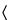905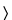UNIFORMITY OF DOSAGE UNITS
This general chapter is harmonized with the corresponding texts of the European Pharmacopoeia and the Japanese Pharmacopoeia. Portions of the general chapter text that are national USP text, and are not part of the harmonized text, are marked with symbols (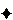) to specify this fact.Note—In this chapter, unit and dosage unit are synonymous.To ensure the consistency of dosage units, each unit in a batch should have a drug substance content within a narrow range around the label claim. Dosage units are defined as dosage forms containing a single dose or a part of a dose of drug substance in each unit. The uniformity of dosage units specification is not intended to apply to suspensions, emulsions, or gels in unit-dose containers intended for external, cutaneous administration.
The term “uniformity of dosage unit” is defined as the degree of uniformity in the amount of the drug substance among dosage units. Therefore, the requirements of this chapter apply to each drug substance being comprised in dosage units containing one or more drug substances, unless otherwise specified elsewhere in this Pharmacopeia.
The uniformity of dosage units can be demonstrated by either of two methods, Content Uniformity orWeightVariation (see Table 1). The test for Content Uniformity of preparations presented in dosage units is based on the assay of the individual content of drug substance(s) in a number of dosage units to determine whether the individual content is within the limits set. The Content Uniformity method may be applied in all cases.
The test forWeightVariation is applicable for the following dosage forms:
 (W1) Solutions enclosed in unit-dose containers and into soft capsules; (W2) Solids (including powders, granules, and sterile solids) that are packaged in single-unit containers and contain no active or inactive added substances; (W3) Solids (including sterile solids) that are packaged in single-unit containers, with or without active or inactive added substances, that have been prepared from true solutions and freeze-dried in the final containers and are labeled to indicate this method of preparation; and (W4) Hard capsules, uncoated tablets, or film-coated tablets, containing 25 mg or more of a drug substance comprising 25% or more, by weight, of the dosage unit or, in the case of hard capsules, the capsule contents, except that uniformity of other drug substances present in lesser proportions is demonstrated by meeting the requirements for Content Uniformity.
The test for Content Uniformity is required for all dosage forms not meeting the above conditions for theWeightVariation test.1
Table 1. Application of Content Uniformity (CU) and Weight Variation (WV) Tests for Dosage Forms
Dosage Form Type Subtype Dose & Ratio of
Drug Substance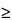25 mg and25% <25 mg or <25%
Tablets Uncoated   WV CU
Coated Film WV CU
Others CU CU
Capsules Hard   WV CU
Soft Suspension, emulsion, or gel CU CU
Solutions WV WV
Solids in
single-unit containers
Single component   WV WV
Multiple components Solution freeze-dried in
final container
WV WV
Others CU CU
Solutions in unit-dose containersand into soft capsulesWV WV
Others     CU CU

CONTENT UNIFORMITY
Select not fewer than 30 units, and proceed as follows for the dosage form designated.
Where different procedures are used for assay of the preparation and for the Content Uniformity test, it may be necessary to establish a correction factor to be applied to the results of the latter.
Solid Dosage Forms
Assay 10 units individually using an appropriate analytical method. Calculate the acceptance value (see Table 2).
Liquid or Semi-Solid Dosage Forms
Assay 10 units individually using an appropriate analytical method. Carry out the assay on the amount of well-mixed material that is removed from an individual container in conditions of normal use, and express the results as delivered dose. Calculate the acceptance value (see Table 2).
Calculation of Acceptance Value
Calculate the acceptance value by the formula:
in which the terms are as defined in Table 2.
Table 2
Variable Definition Conditions Value
X Mean of individual contents (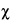1,2, …,n), expressed as a percentage of the label claim1,2, …,n Individual contents of the units tested, expressed as a percentage of the label claim
n Sample size (number of units in a sample)
k Acceptability constant If n = 10, then k = 2.4
If n = 30, then k = 2.0
s Sample standard deviation
RSD Relative standard deviation (the sample standard deviation expressed as a percentage of the mean)   100s/X
M (case 1) to be applied when
T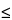101.5
Reference value If 98.5%X101.5%, then M = X (AV = ks)
If X <98.5%, then M = 98.5%
(AV = 98.5 – X + ks)
If X >101.5%, then M = 101.5%
(AV = X – 101.5 + ks)
M (case 2) to be applied when
T >101.5
Reference value If 98.5XT, then M = X
(AV = ks)
If X <98.5%, then M = 98.5%
(AV = 98.5 – X + ks)
If X >T, then M = T%
(AV = X – T + ks)
Acceptance value (AV)     General formula:

(Calculations are specified above for the different cases.)
L1 Maximum allowed acceptance value   L1 = 15.0 unless otherwise specified
L2 Maximum allowed range for deviation of each dosage unit tested from the calculated value of M On the low side, no dosage unit result can be less than [1–(0.01)(L2)]M, while on the high side, no dosage unit result can be greater than [1 + (0.01)(L2)]M. (This is based on an L2 value of 25.0.) L2 = 25.0 unless otherwise specified
T Target content per dosage unit at the time of manufacture, expressed as a percentage of the label claim. Unless otherwise stated, T is 100.0%, or T is the manufacturer's approved target content per dosage unit.WEIGHTVARIATION
Carry out an assay for the drug substance(s) on a representative sample of the batch using an appropriate analytical method. This value is result A, expressed as percentage of label claim (see Calculation of Acceptance Value). Assume that the concentration (weight of drug substance per weight of dosage unit) is uniform. Select not fewer than 30 dosage units, and proceed as follows for the dosage form designated.
Uncoated or Film-Coated Tablets
Accurately weigh 10 tablets individually. Calculate the content, expressed as percentage of label claim, of each tablet from theweightof the individual tablet and the result of the Assay. Calculate the acceptance value.
Hard Capsules
Accurately weigh 10 capsules individually, taking care to preserve the identity of each capsule. Remove the contents of each capsule by a suitable means. Accurately weigh the emptied shells individually, and calculate for each capsule the netweightof its contents by subtracting theweightof the shell from the respective grossweight. Calculate the drug substance content of each capsule from thenet weightof the individual capsulecontentand the result of the Assay. Calculate the acceptance value.
Soft Capsules
Accurately weigh 10 intact capsules individually to obtain their grossweights, taking care to preserve the identity of each capsule. Then cut open the capsules by means of a suitable clean, dry cutting instrument such as scissors or a sharp open blade, and remove the contents by washing with a suitable solvent. Allow the occluded solvent to evaporate from the shells at room temperature over a period of about 30 minutes, taking precautions to avoid uptake or loss of moisture. Weigh the individual shells, and calculate the net contents. Calculate the drug substance content in each capsule from theweightof product removed from the individual capsules and the result of the Assay. Calculate the acceptance value.
Solid Dosage Forms Other Than Tablets and Capsules
Proceed as directed for Hard Capsules, treating each unit as described therein. Calculate the acceptance value.
Liquid Dosage Forms
Accurately weigh the amount of liquid that is removed from each of 10 individual containers in conditions of normal use. If necessary, compute the equivalent volume after determining the density. Calculate the drug substance content in each container from the mass of product removed from the individual containers and the result of the Assay. Calculate the acceptance value.
Calculation of Acceptance Value
Calculate the acceptance value as shown in Content Uniformity, except that the individual contents of the units are replaced with the individual estimated contents defined below.1,2, …,n = individual estimated contents of the units tested, wherei = wi × A/W w1, w2, …, wn = individualweightsof the units tested A = content of drug substance (% of label claim) obtained using an appropriate analytical method W = mean of individualweights(w1, w2, …, wn)

CRITERIA
Apply the following criteria, unless otherwise specified.
Solid, Semi-Solid, and Liquid Dosage Forms
The requirements for dosage uniformity are met if the acceptance value of the first 10 dosage units is less than or equal to L1%. If the acceptance value is > L1%, test the next 20 units, and calculate the acceptance value. The requirements are met if the final acceptance value of the 30 dosage units isL1%, and no individual content ofanydosage unit is less than [1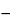(0.01)(L2)]M nor more than [1 + (0.01)(L2)]Mas specifiedin the Calculation of Acceptance Value under Content Uniformity or underWeightVariation. Unless otherwise specified, L1 is 15.0 and L2 is 25.0.

1European Pharmacopoeia and Japanese Pharmacopoeia text not accepted by the United States Pharmacopeia: Alternatively, products listed in item (4) above that do not meet the 25 mg/25% threshold limit may be tested for uniformity of dosage units by Mass Variation instead of the Content Uniformity test if the concentration relative standard deviation (RSD) of the drug substance in the final dosage units is not more than 2%, based on process validation data and development data, and if there has been regulatory approval of such a change. The concentration RSD is the RSD of the concentration per dosage unit (w/w or w/v), where concentration per dosage unit equals the assay result per dosage unit divided by the individual dosage unit weight. See the RSD formula in Table 2.Auxiliary Information— Please check for your question in the FAQs before contacting USP.
Topic/Question Contact Expert Committee
General Chapter William E. Brown
Senior Scientific Liaison
1-301-816-8380
(GCDF2010) General Chapters - Dosage Forms
USP35–NF30 Page 420
Pharmacopeial Forum: Volume No. 35(3) Page 724